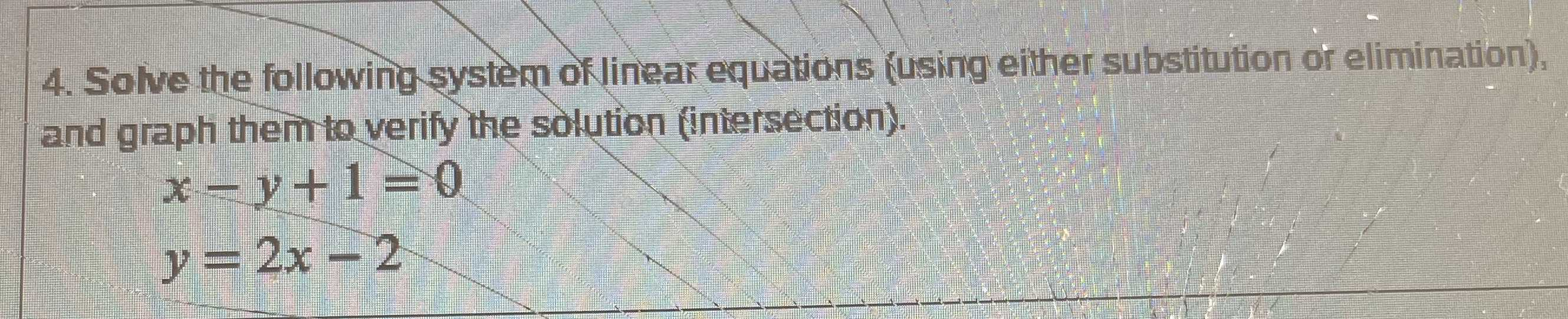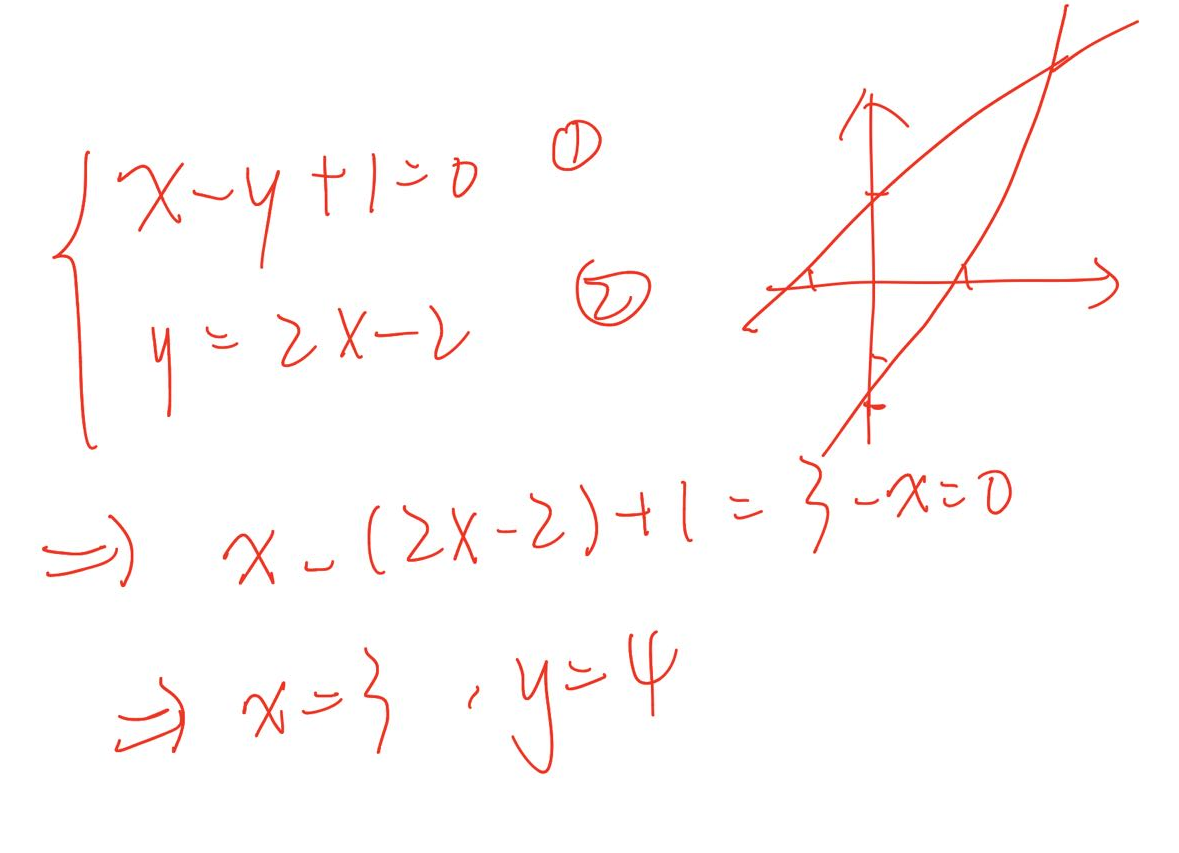### ¿Todavía tienes preguntas de matemáticas?

Pregunte a nuestros tutores expertos
Algebra
Pregunta4. Solve the following system of linear equations (using either substitution or elimination), and graph them to verify the solution (intersection).

$$x - y + 1 = 0$$

$$y = 2 x - 2$$